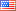# Revision history [back]

### Using the solution of equation

How can I use after solve(eq) the result? For example, the result for this simple linear system
[x + 2y + 4z - 1] [ x + 4z - 5] [ 3x + 6*z - 6] is [[x == -1, y == -2, z == (3/2)]] How can I use x,y,z in an expression, say x^2+y^2+z^2 ?2 Fixed formatting.Mike Hansen4068 ●24 ●48 ●89

### Using the solution of equation

How can I use after solve(eq) the result? For example, the result for this simple linear system

[x + 2y 2*y + 4z 4*z - 1]
[      x + 4z 4*z - 5]
[    3x 3*x + 6*z - 6]


is

[[x == -1, y == -2, z == (3/2)]]


How can I use x,y,z in an expression, say x^2+y^2+z^2 ?3 retagged

### Using the solution of equation

How can I use after solve(eq) the result? For example, the result for this simple linear system

[x + 2*y + 4*z - 1]
[      x + 4*z - 5]
[    3*x + 6*z - 6]


is

[[x == -1, y == -2, z == (3/2)]]


How can I use x,y,z in an expression, say x^2+y^2+z^2 ?4 retagged

### Using the solution of equation

How can I use after solve(eq) the result? For example, the result for this simple linear system

[x + 2*y + 4*z - 1]
[      x + 4*z - 5]
[    3*x + 6*z - 6]


is

[[x == -1, y == -2, z == (3/2)]]


How can I use x,y,z in an expression, say x^2+y^2+z^2 ?